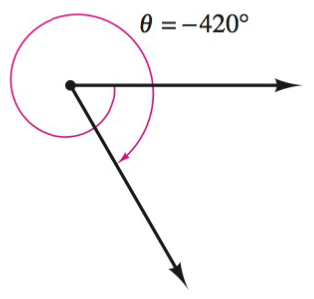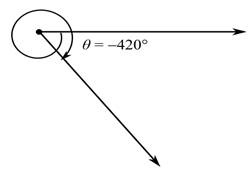Chapter 8.1, Problem 5E### Calculus: An Applied Approach (Min...

10th Edition
Ron Larson
ISBN: 9781305860919

#### Solutions

Chapter
Section### Calculus: An Applied Approach (Min...

10th Edition
Ron Larson
ISBN: 9781305860919
Textbook Problem
1 views

# Finding Coterminal Angles In Exercises 1-6, determine two coterminal angles in degree measure (one positive and one negative) for the angle. See Example 1.To determine

To calculate: The two coterminal angles in degree measure, in which one is positive and another is negative with the help of provided diagram,Explanation

Given Information:

The provided angle is 420°, which is shown below as,

Formula used:

The angle measuring θ has same initial and terminal sides as the angle measuring 360θ, such angles are coterminal angle.

Calculation:

Draw diagram for coterminal angles and provided angle for θ=420°.

From above diagram, let θ+ be the positive coterminal angle and θ be the negative coterminal angle

### Still sussing out bartleby?

Check out a sample textbook solution.

See a sample solution

#### The Solution to Your Study Problems

Bartleby provides explanations to thousands of textbook problems written by our experts, many with advanced degrees!

Get Started

#### In problems 23-58, perform the indicated operations and simplify. 34.

Mathematical Applications for the Management, Life, and Social Sciences

#### Differentiate. F(t)=AtBt2+Ct3

Single Variable Calculus: Early Transcendentals

#### The interval of convergence of is: [−1, 1] [−1, 1) (−1, 1] (−1, 1)

Study Guide for Stewart's Single Variable Calculus: Early Transcendentals, 8th